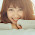## Tuesday, 9 December 2014

### Excel Tip: Calculating Net Present Value (NPV)

Last week we covered the IRR function, which enabled us to calculate the Internal Rate of Return of a project.

This week I am going to introduce the NPV function which allows us to calculate Net Present Value - a function closely related to IRR.

The Net Present Value of a series of cash flows, gives a value of those cash flows today discounted by a required rate of return.
The required rate of return represents represents the investor's time value of money. This is often the rate of return achievable in alternative investments.

So, for example, if we have the following series of cash flows (the same example as used for the IRR post):

Initial outlay  £20,000
Year 1 positive cash flow  £500
Year 2 positive cash flow  £5,000
Year 3 positive cash flow  £8.200
Year 4 positive cash flow  £9,000
Year 5 positive cash flow  £9,000

and we require a 10% annual rate of return.

The syntax of the NPV function is:

=NPV(rate,value1,[value2],.....)

where rate is the required rate of return and value1, value2, etc. are a series of cash flows at the same regular interval as the rate, so if the rate is an annual rate, then the cash flows should be a year apart.

value1 is required, whereas [value2] onwards are optional. These can be entered as values or refer to a range. Note that these cash flows are assumed to be at the end of each period.

So we could write the function for our example as follows:

=NPV(0.1, -20000,500,5000,8200,9000,9000)

which returns £2,257.25.

More likely though, we will have the values held in a range of cells as we did in the IRR example:

B2  -20000
B3   500
B4   5000
B5   8200
B6   9000
B7   9000

In this case our function would be:

=NPV(0.1,B2:B7)

Obviously, we would normally also refer to a cell to provide the rate as well, rather than enter it directly into the formula.

NPV relates to IRR  because IRR is the rate that produces zero NPV, so:

=NPV(IRR(B2:B7),B2:B7)

will always return zero.

If you enjoyed this post, go to the top of the blog, where you can subscribe for regular updates and get two freebies "The 5 Excel features that you NEED to know" and "30 Chants for Better Charts".

1.This calculation or there, I will learn and practice
juegos de autos gratis ,jogos friv

2.Excel is best source to store data & files. I am sharing top essay writing services site.

3.I think I need it. Thank you
friv online
friv2
friv2 2
friv